## Megaminx — Orientation of the Corners of the Last Layer

These are the OCLL cases for a megaminx. The algs are from Erik's site and this site.

•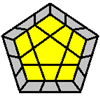CPLL Time!
•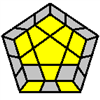(y2') R2' D' R U2 R' D R U2' R
•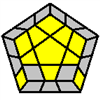(y) L F R' F' L' F R F'
•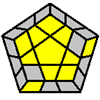F R' F' L F R F' L'
•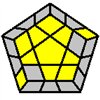(y2') R U2 R' U R U2 R'
•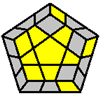R U R' U R U'2 R'
•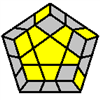(y) R' U' R U' R' U2 R
•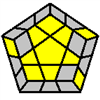(y2') R U2 R' U' R U' R'
•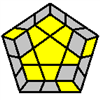(y2) R' U2' R U' R' U R
•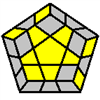R U R' U R U' R' U R U'2 R'
•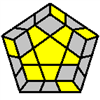R' U2' R U R' U' R U R' U R
•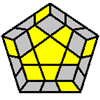R' U2 R U2 R' U2' R U2 R' U R'
•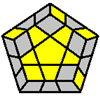(y2) R U R' U R U R' U' R U2' R'
•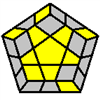R U2 R2' U' R2 U' R2' U2 R
•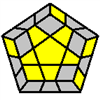(y2) R' U2' R2 U R2' U R2 U2' R'
•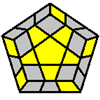(y) R U R' U2 R U2' R' U R U2' R'
•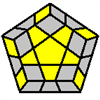(y) R' U' R U2' R' U2 R U' R' U2 R